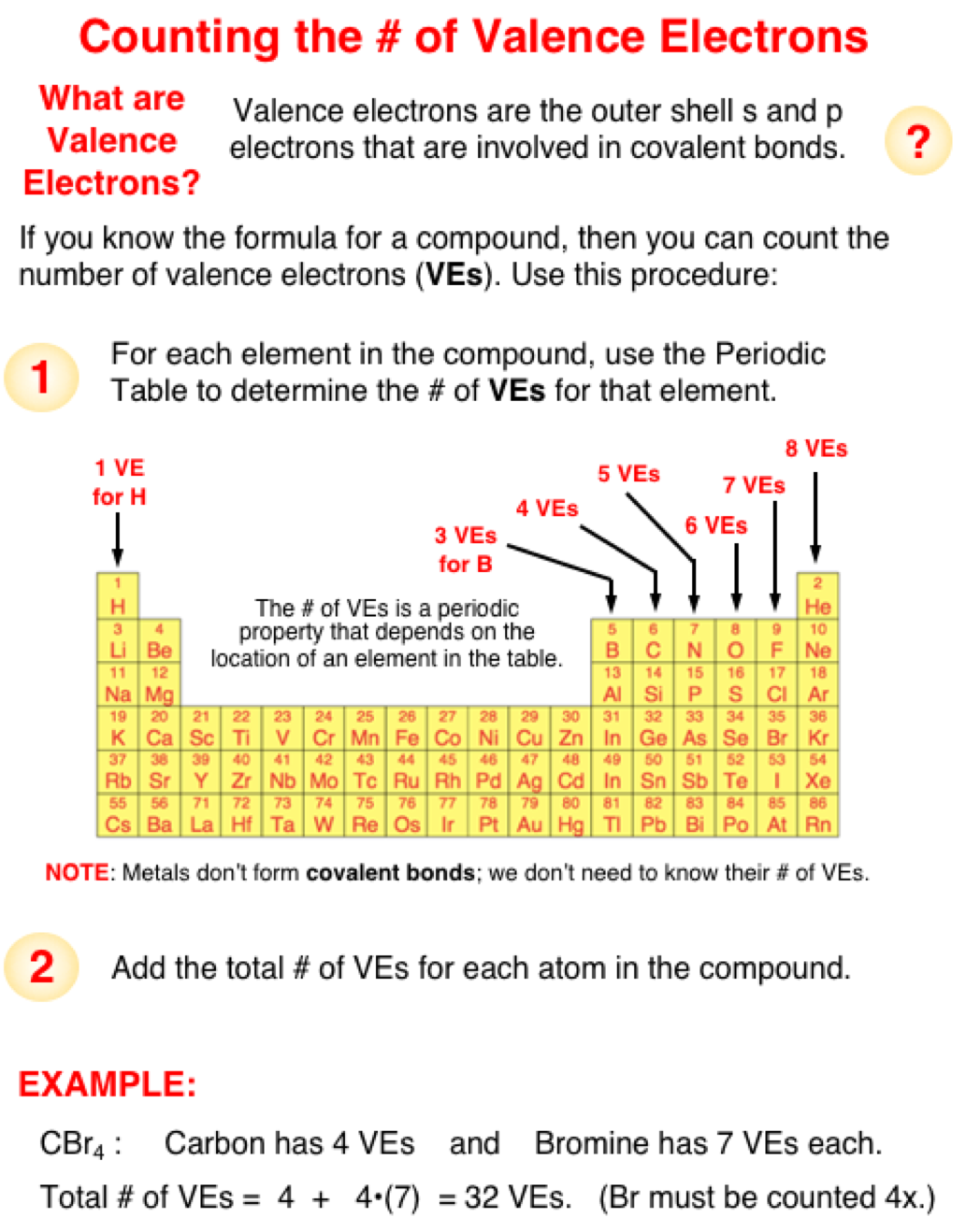#### Counting Valence Electrons

How does one Determine the # of Valence Electrons?
First you need to be able to determine the number of valence electrons present in each element of the compound. Since the number of valence electrons is a periodic property, the value can be easily determined by locating the element in the periodic table. As shown in the graphic below, all Group 1 elements have an s1 electron configuration and thus have one valence electron. All Group 2 elements have an s2 electron configuration and two valenece electrons. Group 13 elements have an s2p1 electron configuration and three valenece electrons.  Similar logic can be applied to all other groups to obtain the results shown in the graphic below.

Once you have determined the number of valence electrons for the atoms of each element in the compound, you are ready to determine the total number of valence electrons in the molecule. To do so, add up all the valence electrons for each atom in the molecule of that compound. For instance, if you are trying to find the total number for valence electrons in CF4, then you will need to first find out that carbon (C) has four valence electrons and fluorine (F) has seven valence electrons. Then add 4 + 7 + 7 + 7 + 7 to get a total of 32 valence electrons. The "7" is added four times since there are four fluorine atoms with 7 valence electrons each.